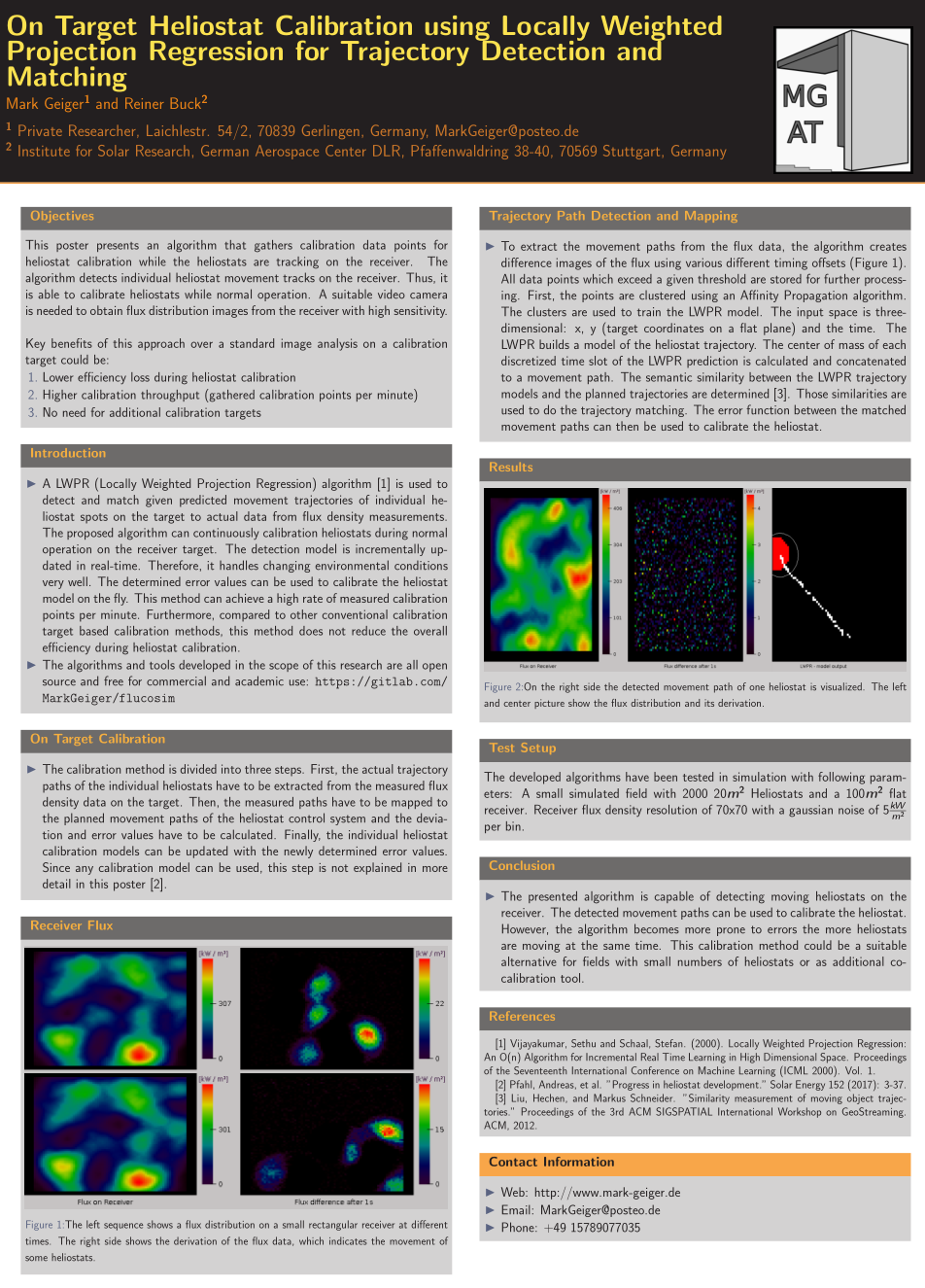## On Target Heliostat Calibration# Simple Power Estimation of Heliostats

A very simple approach of estimating heliostat efficiencies. In this implementation shading and blocking effects are ignored:

``````double PowerEstimation::estimateHeliostatEfficiency(Vector3 &sunVector, const
{
Vector3 position = heliostat.getPos();

// Ray vector from heliostat to AimPoint
Vector3 ray = rayFull.normalize();
Vector3 normalVector = calcHeliostatNormalVector(heliostat, sunVector, target);

// efficiency = shading * cos * reflectivity * blocking * transmission * intercept
double cosine = normalVector.dot(ray); // cos for normalized vectors
double etaBlock = 1.0; // no blocking
double transmission = 0.99326 - 1.046e-4 * rayFull.length() + 1.7e-8 * pow(rayFull.length(), 2)
- 2.845e-12 * pow(rayFull.length(), 3);
double imageDiameter = rayFull.length() * 0.015; // image has diameter of 1.5 m in 100 m distance,
double imageArea = M_PI * pow(imageDiameter/2.0, 2); // assumer circular spot
// area as square of diameter

double etaIntercept = 1;
if (imageArea > areaAperture)
{
etaIntercept = areaAperture / imageArea;
}

return etaShade * cosine * heliostat.getReflectivity() * etaBlock * transmission * etaIntercept;
}
``````

## References

`` Vijayakumar, Sethu & Schaal, Stefan. (2000). Locally Weighted Projection Regression: An O(n) Algorithm for Incremental Real Time Learning in High Dimensional Space. Proceedings of the Seventeenth International Conference on Machine Learning (ICML 2000). Vol. 1.

`` Pfahl, Andreas, et al. "Progress in heliostat development." Solar Energy 152 (2017): 3-37.

`` Liu, Hechen, and Markus Schneider. "Similarity measurement of moving object trajectories." Proceedings of the 3rd ACM SIGSPATIAL International Workshop on GeoStreaming. ACM, 2012.